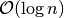# Searching Analysis¶

Consider a finite collection of element. Finding whether element exsists in collection is known as Searching. Following are some of the comparision based Searching Algorithms.

• Linear Search
• Binary Search

Before looking at the analysis part, we shall examine the Language in built methods to searching

## The in operator and list.index()¶

We have already seen the in operator in several contexts. Let’s see the working of in operator again

In :

x = list(range(10))

In :

x

Out:

[0, 1, 2, 3, 4, 5, 6, 7, 8, 9]

In :

6 in x

Out:

True

In :

100 in x

Out:

False

In :

x.index(6)

Out:

6

In :

x.index(100)

---------------------------------------------------------------------------
ValueError                                Traceback (most recent call last)
<ipython-input-6-0f87238c301b> in <module>()
----> 1 x.index(100)

ValueError: 100 is not in list


## Standard import statement¶

In :

from openanalysis.searching import SearchingAlgorithm,SearchAnalyzer
%matplotlib inline
%config InlineBackend.figure_formats={"svg", "pdf"}


SearchingAlgorithm is the base class providing the standards to implement searching algorithms, SearchAnalyzer analyses the algorithm

## SearchingAlgorithm class¶

Any searching algorithm, which has to be implemented, has to be derived from this class. Now we shall see data members and member functions of this class.

### Data Members¶

• name - Name of the Searching Algorithm
• count - Holds the number of basic operations performed

### Member Functions¶

• __init__(self, name): - Initializes algorithm with a name
• search(self, array, key): _ The base searching function. Sets count to 0. array is 1D numpy array,key is the key of element to be found out

## SearchAnalyzer class¶

This class provides the visualization and analysis methods. Let’s see its methods in detail

• __init__(self, searcher): Initializes visualizer with a Searching Algorithm.
• searcher is a class, which is derived from SearchingAlgorithm
• analyze(self, maxpts=1000):
• Plots the running time of searching algorithm by searching in 3 cases
• Key is the first element, Key is the last element, Key at random index
• Analysis is done by inputting sorted integer arrays with size staring from 100, and varying upto maxpts in the steps of 100, and counting the number of basic operations
• maxpts Upper bound on size of elements chosen for analysing efficiency
In :

bin_visualizer = SearchAnalyzer(BinarySearch)

<matplotlib.figure.Figure at 0x7ff57329b978>

In :

bin_visualizer.analyze(progress=False)

Please wait while analyzing Binary Search AlgorithmNoteperformance in all cases

## compare(algs)¶

algs is a list of classes derived from SearchingAlgorithm. It performs tests and plots the bar graph comapring the number of basic operations performed by each algorithm.

## Example File¶

You can see more examples at Github